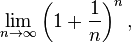e’s are good – He’s Leonard Euler.

Having recently starting a topic on the exponential function, I was really struggling to find some good resources online – which is pretty surprising given that e is one of the most important and useful numbers in mathematics. So, here are some possible approaches.

1) e memorisation challenge.

This is always surprisingly popular – and a great starter which reinforces both that e is infinite and also that it’s just a number – so shouldn’t be treated like other letters when it comes to calculus.

5 minutes: How many digits of e can students remember?

Recital at the front. You can make this easier by showing them that  2. 7 1828 1828 45 90 45  they only need to remember 2.7 and then that 1828 repeated, followed by the angles in a triangle – 45, 90, 45.  Good students can get 20 places plus – and for real memory champions here are the first 1000 digits .

2) Introduction to Leonard Euler

Euler is not especially well known outside of mathematics, yet is undoubtedly one of the true great mathematicians. As well as e being named after him (Euler’s number), he published over 800 mathematical papers on everything from calculus to number theory to algebra and geometry.

30-40 minutes – The Seven Bridges of Königsberg
This is one of Euler’s famous problems – which he invented a whole new branch of mathematics (graph theory) to try and solve. Here is the problem:

The city of Königsberg used to have seven bridges across the river, linking the banks with two islands. The people living in Königsberg had a game where they would try to walk across each bridge once and only once. You can chose where to start – but you must cross each bridge only once:The above graphic is taken from the Maths is Fun resource on Euler’s bridge problem.  It’s a fantastically designed page – which takes students through their own exploration of how to solve similar problems (or as in the case of the 7 bridges problem, understanding why it has no solution).

30 minutes – why e ?

This is a good activity for students learning about differentiation for the first time.

First discuss exponential growth (example the chessboard and rice problem ) to demonstrate how rapidly numbers grow with exponential growth – ie. if I have one grain of rice on the first square, two on the second, how many will I have on the 64th square?

Next, students are given graph paper and need to sketch  y = 2^x y = e^x y = 3^x for between x = 0 and 3.  Students can see that y = e^x is between y=2^x and y = 3^x on the graph, so why is e so much more useful than these numbers?   By graphical methods they should find the gradient when the graphs cross the y axis.    Look at how the derivative of e^x is still e^x – which makes it really useful in calculus.   This is a nice short video which explains graphically why e was chosen to be 2.718…

4) The beauty of e.

10-30 minutes (depending on ability), discussion of some of the beautiful equations associated with e and Euler:

a) Euler Identity – frequently voted the most beautiful equation of all time by mathematicians, it links 5 of the most important constants in mathematics together into a single equation.b) e as represented as a continued infinite fraction (can students spot the pattern? – the LHS is given by 2 then 1,2,1  1,4,1  1,6,1 etc.c) e as the infinite sum of factorials:d) e as the limit:So, hopefully that should give some ideas for looking at this amazing number. (The post title will be lost on anyone not a teenager in England in the 1990s -to find out what you’re missing out on, here’s the song).

If you enjoyed this topic you may also like:

Cesaro Summation: Does 1 – 1 + 1 – 1 … = 1/2? – a post which looks at the maths behind this particularly troublesome series.

A Maths Snooker Puzzle – a great little puzzle which tests logic skills.

IB RevisionIf you’re already thinking about your coursework then it’s probably also time to start planning some revision, either for the end of Year 12 school exams or Year 13 final exams. There’s a really great website that I would strongly recommend students use – you choose your subject (HL/SL/Studies if your exam is in 2020 or Applications/Analysis if your exam is in 2021), and then have the following resources:The Questionbank takes you to a breakdown of each main subject area (e.g. Algebra, Calculus etc) and each area then has a number of graded questions. What I like about this is that you are given a difficulty rating, as well as a mark scheme and also a worked video tutorial.  Really useful!The Practice Exams section takes you to ready made exams on each topic – again with worked solutions.  This also has some harder exams for those students aiming for 6s and 7s and the Past IB Exams section takes you to full video worked solutions to every question on every past paper – and you can also get a prediction exam for the upcoming year.

I would really recommend everyone making use of this – there is a mixture of a lot of free content as well as premium content so have a look and see what you think.